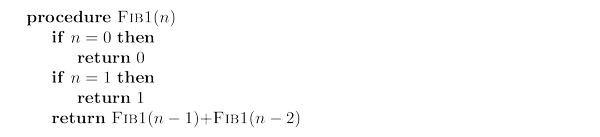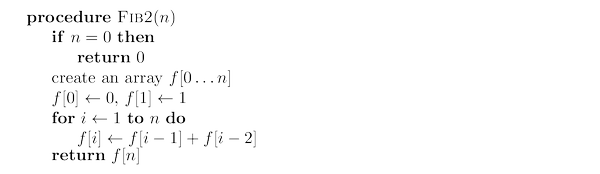# Fibonacci Numbers solved by 5558

Feb. 21, 2014, 3:28 p.m. by Rosalind Team

## Fibonacci numbers

The Fibonacci numbers grow almost as fast as the powers of $2$: for example, $F_{30}$ is over a million, and $F_{100}$ is already $21$ digits long! In general, $F_n \approx 2^{0.694n}$. No other sequence of numbers has been studied as extensively, or applied to more fields: biology, demography, art, architecture, music, to name just a few. And, together with the powers of $2$, it is computer science’s favorite sequence.

## Problem

The Fibonacci numbers $0, 1, 1, 2, 3, 5, 8, 13, 21, 34, \dots$ are generated by the following simple rule $$F_n = \begin{cases} F_{n-1}+F_{n-2}, & n > 1\, ,\\ 1, & n=1 \, ,\\ 0, & n=0 \, .\\ \end{cases}$$

Given: A positive integer $n \le 25$.

Return: The value of $F_n$.

## Sample Dataset

6


## Sample Output

8


## DiscussionFigure 1. A tree showing the branching, exponential nature of recursive calls in fib1. The bottom row of the tree has calls; once the row grows to depth 20, there are 1,048,576 recursive calls.

One idea is to slavishly implement the recursive definition of $F_n$. Here is the pseudocode of the resulting algorithm:Let $T(n)$ be the number of steps needed to compute ${\tt fib1}(n)$; what can we say about this function? For starters, if $n \leq 2$, then the procedure halts almost immediately, after just a couple of steps. Therefore, $T (n) \le 2$ for $n ≤ 1$. For larger values of $n$, there are two recursive calls of ${\tt fib1}$, taking respective times $T(n−1)$ and $T(n−2)$, plus three computer steps (checks on the value of $n$ and a final addition). Therefore, $$T (n) = T (n − 1) + T (n − 2) + 3 \text{ for } n > 1.$$ Compare this to the recurrence relation for $F_n$: we immediately see that $T(n) \ge F_n$.

This is very bad news: the running time of the algorithm grows as fast as the Fibonacci numbers themselves! $T(n)$ is exponential in $n$, which implies that the algorithm is impractically slow except for very small values of $n$.

Let’s try to understand why ${\tt fib1}$ is so slow. Figure 1 shows the cascade of recursive invocations triggered by a single call to ${\tt fib1}(n)$. Notice that many computations are repeated! A more sensible scheme would store the intermediate results—the values $F_0, F_1, \dots, F_{n−1}$—as soon as they become known.As with ${\tt fib1}$, the correctness of this algorithm is self-evident because it directly uses the definition of $F_n$. How long does it take? The inner loop consists of a single computer step and is executed $n − 1$ times. Therefore the number of computer steps used by ${\tt fib2}$ is linear in $n$. From exponential we are down to polynomial, a huge breakthrough in running time. It is now perfectly reasonable to compute $F_{200}$ or even $F_{200\,,000}$.

## Visualization

To better feel the difference between polynomial and exponential time, try to compute $F_{20}$ by using the following visualization by David Galles: http://www.cs.usfca.edu/~galles/visualization/DPFib.html. Selecting "Fibonacci Recursive" shows the enormous number of recursive calls that the program tries to make in ${\tt fib1}$. Stop the animation when you get tired of watching it, and instead click "Fibonacci Table" to see just how much quicker using ${\tt fib2}$ is. (Note however that a slightly different definition is used there: $F_0=F_1=1$.)